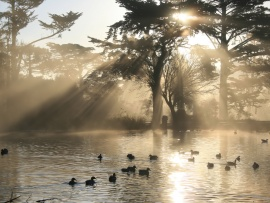WE USE COOKIES           ** FACEBOOK PAGE
This scenic wallpaper shows the sun's rays coming around a tree. Other rays reflect off the water of the lake. These types of rays are known as Crepuscular rays, which are rays of sunlight that appear to radiate from a point in the sky and stream through cloud gaps and other objects like trees in a forest. This image was taken at Stow Lake in Golden Gate Park, San Francisco. Image by Mila Zinkova.## Download Rays of Sun wallpaper | Leave a Comment

### function roundNumber(b,c){var a=Math.round(b*Math.pow(10,c))/Math.pow(10,c);return a}function resPrints(){var b=screen.width;var c=screen.height;var a=roundNumber(b/c,2);var d="Your display Resolution: <strong> "+b+" x "+c+" </strong> | Aspect Ratio:<strong> ";if(a==1.25||a==1.33){d+="Standard 4:3"}else{if(a==1.6||a==1.67){d+="Wide 8:5"}else{if(a==1.78){d+="HD 16:9"}else{if(a==1.76){d+="Mobile PSP"}else{if(a==0.75||(a==1.33&&b<=640)){d+="Mobile VGA"}else{if(a==0.6||(a==1.67&&b<=800)){d+="Mobile WVGA"}else{if(a==0.67||(a==1.5&&b==480)){d+="Mobile iPhone"}else{if(a==0.8){d+="Mobile Phone"}else{d="Unknown"}}}}}}}}d+="</strong>";document.write(d)}; resPrints();

Wallpaper details:
Added: Monday, 04 October 2010 15:46
Downloads: 39684
Sub Categories:
License: Free for personal wallpaper use only